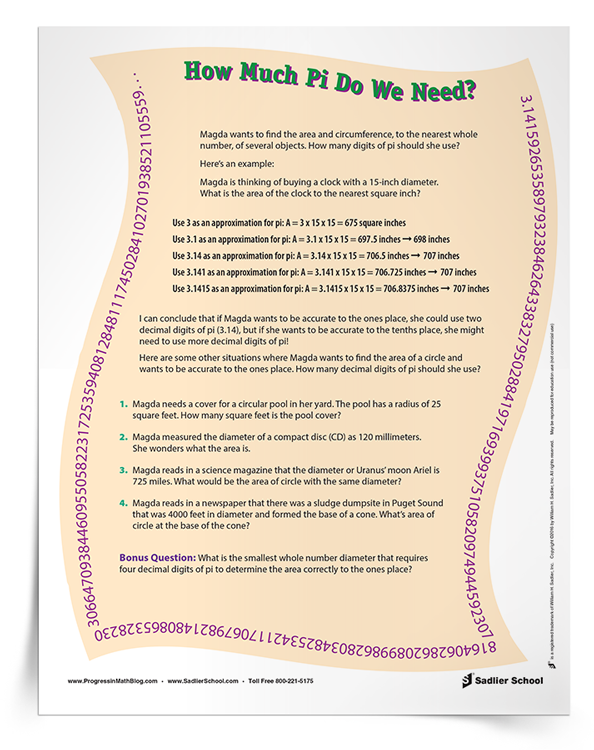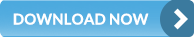1.800.221.5175
Core Program
Full Access
Core Program
Full Access
Core Program
Full Access
National Program
Full Access
New York
Critical Thinking for Active Math Minds
Preparing for Standards Based Assessments
Vocabulary Workshop, Tools for Comprehension Grades 1–5
Print Program
Interactive Edition
Print Program
Interactive Edition
Vocabulary Workshop, Tools for Excellence Grades 6–12+
Print Program
Interactive Edition
Vocabulary for Success
Progress English Language Arts Grades K–8
National Program
Full Access
New York
Grammar Workshop, Tools for Writing
Grammar for Writing
Writing a Research Paper
Writing Workshop
Print Program
Interactive Practice Bundle
Fluency Booster Practice Book
Interactive Assessments
Print Program
Interactive Edition

A K–8 resource to support deep comprehension of math skills and conceptsTopics:

#### July 1, 2016 6-8-the-number-system

Developing number sense for students in the upper middle grades includes their understanding the difference between rational and irrational numbers. Irrational numbers have at times been controversial in the development of mathematics and can provide a great opportunity to tie math concepts in with history.Irrational numbers first receive formal treatment in Grade 8 in the Common Core Standards for Mathematics. However, the geometric ideas that lead to the development of irrational numbers (circumference, area of a circle, area and side lengths of squares) are part of the middle school mathematics curriculum.

Of course, rational numbers should be introduced as numbers that can be expressed as the ratio of two integers a/b, where b ≠ 0. Even as this definition is developed, it is helpful for students to know that there are numbers that aren’t rational! This doesn’t mean that they need to master the definition of irrational (or non-rational) numbers, but it can pique their appetite for something that is coming in future grades. Developing number sense in this area — that there are different number types that they will learn about as they progress as mathematical thinkers — is an important concept for students to absorb.

The activities will help students with skills that will become more important over time, culminating with their study of irrational numbers in Grade 8. These number sense activities build upon middle-grade work on area of squares and circles. These three activities will help students develop a sense of irrational numbers without giving a formal definition. Download the “How Much Pi Do We Need?” activity to use in your class today!

## Activity 1: Estimating the Value of Pi

One way to estimate pi is to have students draw, either by using a compass or by tracing, a circle on a sheet of paper. They can use string to outline the circumference of the circle, then measure the length of the string (I suggest measuring in milimeters or centimeters). Students can then use a ruler to find the diameter of the circle. Pi’s common definition is the ratio of the circumference, where the circumference is divided by the diameter to estimate value of pi. Have students find the value of pi using a calculator or long division. Ask them to share their results. If students are careful with their calculations, they will see that their results are all close to 3. This is a great time to discuss a little bit of the history of the approximation of pi — easily found on Wikipedia — so that students can make a connection to the history of various cultures and to consider how long human cultures have been pursuing the exact value of this ratio now known as pi.

Talk with your history teacher colleagues about possible tie-ins to Chinese, Babylonian, Greek, East Indian, Arabic, or Egyptian cultures that they may be studying. As students discuss how ancient cultures needed pi and wanted to know its exact values, they will be developing number sense for themselves as well as seeing that numbers have a cultural component, not just a detached numerical value.

This activity can culminate by showing students how to calculate pi to several digits and discussing how close their estimates can be to the actual number.

A related activity would be to have students draw a circle on a sheet of graph paper and count the squares (and parts of squares) in order to estimate the area of the circle. Dividing their estimate by the square of the radius will also yield approximations for pi.

## Activity 2: How Much Pi Do We Need?

Students’ concept of pi is sometimes limited by the kinds of problems we give them. Many of my students think of pi as 3.14, which is a very common estimate for pi — one which is often used on standardized tests. However, the goal of this activity is developing number sense. In this case, we want to have students see how many digits of pi are needed in order to get an answer accurately rounded to the ones place.Download this activity and encourage students to use a calculator to complete it. That way, the focus is on seeing the effect of using varying estimates for pi rather than on practicing multi-digit decimal multiplication. You can have a great discussion in class about how much accuracy is needed for everyday work as opposed to scientific work.

## Activity 3: Finding the Length of a Side of a Square

This activity will help students begin the important task of developing number sense about the value of square roots. This doesn’t need to be a formal introduction to square roots, as the idea is presented geometrically.

Introduce the activity using squares with areas that have perfect square values such as 9 square feet or 25 square centimeters. Ask students identify the length of the square. Then ask them how they would check their response (by multiplying the value of the side length times itself).

After students are comfortable with this line of questioning and you feel that they are attuned to this idea, ask, “If a square has an area of 8 square inches, what is the length of one side of the square?” Three inches is too big, and two inches is too small. Some students will think it is not possible to find the answer. But some students will come to the conclusion that the side length must be between two and three.

This is where the fun begins. Many students will guess that the length is 2.5 inches. Ask them how they can check their answer. They may try by multiplying 2.5 x 2.5 = 6.25. This value is too small. Ask students to try different values to see who can get the closest. The closest one-digit decimal approximation is 2.8, but it is not exact. You can ask students what they think they should do about this. Trying two-digit decimals (then three, then four digits) would achieve results that are closer and closer to eight. As the decimals get longer, you will find that using calculators is important.

While some students will tire of this, others will be determined to find the exact answer. However, you will need to explain that there isn’t one, because the square root of eight is irrational.

Here are many digits of the square root of 8: 2.8284271247461900976033774484193961571393437507538961463533594759814649569242…

You can see over a million digits of this number on the NASA website.

Getting students to begin to understand that there are certain numbers that can’t be exactly determined in decimal notation is a difficult and important idea in developing number sense that will help them in high school, college, and beyond.